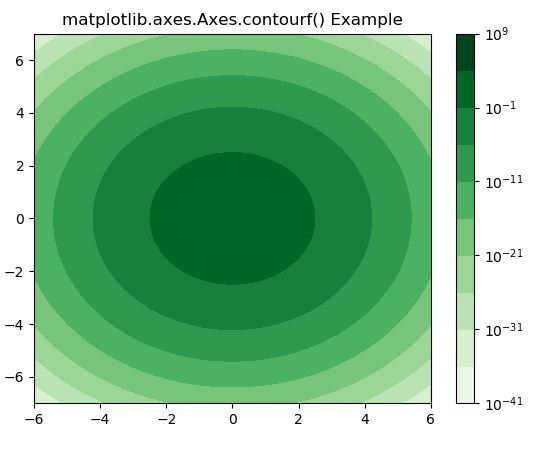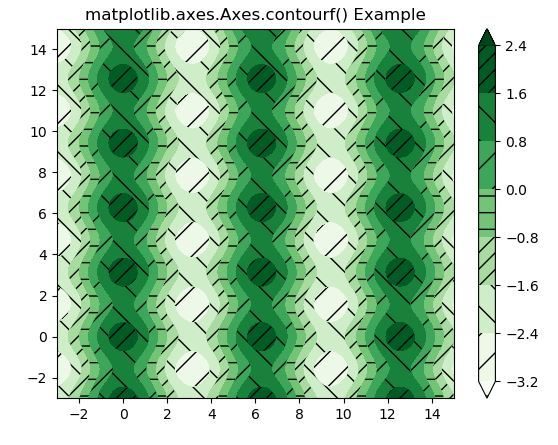Skip to content
Related Articles
Matplotlib.axes.Axes.contourf() in Python
• Last Updated : 13 Apr, 2020

Matplotlib is a library in Python and it is numerical – mathematical extension for NumPy library. The Axes Class contains most of the figure elements: Axis, Tick, Line2D, Text, Polygon, etc., and sets the coordinate system. And the instances of Axes supports callbacks through a callbacks attribute.

## matplotlib.axes.Axes.contourf() Function

The Axes.contourf() function in axes module of matplotlib library is also used to plot contours. But contourfdraw filled contours, while contourf draws contour lines.

Syntax:

`Axes.contourf(self, *args, data=None, **kwargs)`

Parameters: This method accept the following parameters that are described below:

• X, Y: These parameter are the coordinates of the values in Z.
• Z : This parameter is the height values over which the contour is drawn.
• levels : This parameter is used to determine the numbers and positions of the contour lines / regions.

Returns: This returns the following:

• c :This returns the QuadContourSet.

Below examples illustrate the matplotlib.axes.Axes.contourf() function in matplotlib.axes:

Example-1:

 `# Implementation of matplotlib function``import` `numpy as np``import` `matplotlib.pyplot as plt``from` `numpy ``import` `ma``from` `matplotlib ``import` `ticker, cm`` ` `N ``=` `1000``x ``=` `np.linspace(``-``6.0``, ``6.0``, N)``y ``=` `np.linspace(``-``7.0``, ``7.0``, N)``X, Y ``=` `np.meshgrid(x, y)`` ` `Z1 ``=` `np.exp(``-``(X)``*``*``2` `-` `(Y)``*``*``2``)``z ``=` `50` `*` `Z1``z[:``5``, :``5``] ``=` `-``1``z ``=` `ma.masked_where(z <``=` `0``, z)`` ` `fig, ax ``=` `plt.subplots()``cs ``=` `ax.contourf(X, Y, z, locator ``=` `ticker.LogLocator(),``                 ``cmap ``=``"Greens"``)`` ` `cbar ``=` `fig.colorbar(cs)``ax.set_title(``'matplotlib.axes.Axes.contourf() Example'``)`` ` `plt.show()`

Output:Example-2:

 `# Implementation of matplotlib function``import` `matplotlib.pyplot as plt``import` `numpy as np`` ` `# invent some numbers, turning the``# x and y arrays into simple 2d arrays,``# which make combining them together easier.``x ``=` `np.linspace(``-``3``, ``15``, ``450``).reshape(``1``, ``-``1``)``y ``=` `np.linspace(``-``3``, ``15``, ``720``).reshape(``-``1``, ``1``)``z ``=` `np.cos(x)``*``2` `-` `np.sin(y)``*``*``2`` ` `# we no longer need x and y to be``# 2 dimensional, so flatten them.``x, y ``=` `x.flatten(), y.flatten()`` ` `fig1, ax1 ``=` `plt.subplots()``cs ``=` `ax1.contourf(x, y, z, hatches ``=``[``'-'``, ``'/'``, ``'\\', '``/``/``'],``                  ``cmap ``=``'Greens'``, extend ``=``'both'``, alpha ``=` `1``)``fig1.colorbar(cs)``ax1.set_title(``'matplotlib.axes.Axes.contourf() Example'``)``plt.show()`

Output:Attention geek! Strengthen your foundations with the Python Programming Foundation Course and learn the basics.

To begin with, your interview preparations Enhance your Data Structures concepts with the Python DS Course. And to begin with your Machine Learning Journey, join the Machine Learning – Basic Level Course

My Personal Notes arrow_drop_up